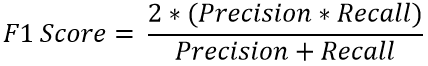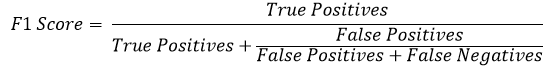Classification

Logistic Regression

Machine Learning

January 7, 2021

# What is F1 score?

We know that for unbalanced datasets we can’t rely on accuracy. Here we have to measure the performance of the model using Precision & Recall. But the question arises, how much Precision and how much Recall. Since Precision and Recall share an inverse relationship, we can’t have the ideal scenario where both Precision & Recall are high. We need a metric that quantifies the balance between precision and recall. A metric which could tell us if the Precision Recall Tradeoff is optimum or not. This where F1 score comes in, it is the harmonic mean of Precision & Recall.It is mostly used for unbalanced datasets and acts as a substitute for accuracy metric.by : Monis Khan

Quick Summary:

We know that for unbalanced datasets we can’t rely on accuracy. Here we have to measure the performance of the model using Precision & Recall. But the question arises, how much Precision and how much Recall. Since Precision and Recall share an inverse relationship, we can’t have the ideal scenario where both Precision & Recall […]